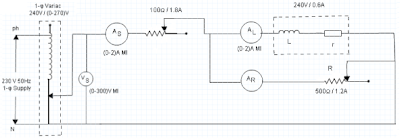# Measurement of Choke Coil Parameters by Using 3-Ammeter Method

Our aim here is to measure the inductance and inherent resistance of a given choke coil and also to determine the quality factor using 3-Ammeter method.

Equivalent circuit of any choke coil consists of inductance in addition to inherent resistance. Usually, any given choke coil is represented by a pure inductance (L) in series with equivalent resistance (r). The equivalent resistance takes into effect the iron losses in the core of choke coil and its inherent resistance as well. By using 3-Ammeter method and 3-Voltmeter method we can efficiently measure these two parameters.

The circuit diagram for the measurement of choke coil parameters for 3-Ammeter method is as given below:

#####3-Ammeter Method

In this method 3-ammeters are used, one of them measure current supplied by the source to the parallel combination of choke coil and external resistance. The remaining two ammeters measure the current through choke coil and resistor.

The vector diagram for the 3-ammeter method is as shown hereunder along with necessary formula for finding coil resistance, coil impedance, coil reactance, Q-factor and also the power absorbed by the choke coil.

### Procedure for 3-Ammeter method of measuring choke coil parameters :-

1. Give the connections as in the circuit diagram.
2. Apply 230V, 1-φ supply to the parallel combination of choke coil and external resistance.
3. Note down the reading of all the three ammeters.
4. Now vary the rheostat (500Ω/1.8A) so that Is varies in suitable steps.
5. At each step tabulate the reading of the three ammeters and calculate coil resistance and inductance using the formula given above.
6. Also determine Q-factor in each step and compare the results.
One Response